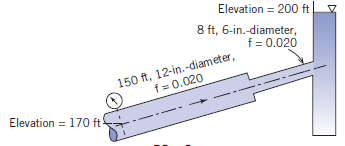Problem

# Water is flowing. Calculate the gage reading when the velocity in the 12-in.-diameter pipe...

Water is flowing. Calculate the gage reading when the velocity in the 12-in.-diameter pipe is 8 ft/s.#### Step-by-Step Solution

Solution 1

Calculate the velocity in the 6 in diameter pipe using continuity equation.

\begin{aligned} &A_{1} V_{1}=A_{2} V_{2} \\ &V_{1}=\frac{A_{2}}{A_{1}} V_{2} \end{aligned}

Here, $$A_{1}$$ is the area of 12 in diameter pipe and $$A_{2}$$ is the area of 6 in diameter pipe.

Substitute $$8 \mathrm{ft} / \mathrm{s}$$ for $$V_{1},\left(\frac{\pi}{4} \times 12^{2}\right)$$ in $$^{2}$$ for $$A_{1},\left(\frac{\pi}{4} \times 6^{2}\right)$$ in $$^{2}$$ for $$A_{2}$$.

\begin{aligned} V_{2} &=\frac{\left(\frac{\pi}{4} \times 12^{2}\right)}{\left(\frac{\pi}{4} \times 6^{2}\right)} \times 8 \\ &=32 \mathrm{ft} / \mathrm{s} \end{aligned}

Calculate the head loss in the pipe with 12 in diameter.

$$H_{a}=f_{1} \frac{L_{1}}{D_{1}} \frac{V_{1}^{2}}{2 g}$$

Here, $$L_{1}$$ is the length of the pipe, $$g$$ is the acceleration due to gravity, $$D_{1}$$ is the diameter of the pipe and $$f_{1}$$ is the friction factor.

Substitute $$0.02$$ for $$f_{1}, 150 \mathrm{ft}$$ for $$L_{1}, 32.2 \mathrm{ft} / \mathrm{s}^{2}$$ for $$g,(12 / 12) \mathrm{ft}$$ for $$D_{1}, 8 \mathrm{ft} / \mathrm{s}$$ for $$V_{1}$$.

\begin{aligned} H_{n} &=0.02 \times \frac{150}{(12 / 12)} \times \frac{8^{2}}{2 \times 32.2} \\ &=2.98 \mathrm{ft} \end{aligned}

Calculate the head loss in the pipe with 6 in diameter.

$$H_{/ 2}=f_{2} \frac{L_{2}}{D_{2}} \frac{V_{2}^{2}}{2 g}$$

Here, $$L_{2}$$ is the length of the pipe, $$D_{2}$$ is the diameter of the pipe, $$f_{2}$$ is the friction factor.

Substitute $$0.02$$ for $$f_{2}, 8 \mathrm{ft}$$ for $$L_{2}, 32.2 \mathrm{ft} / \mathrm{s}^{2}$$ for $$g,(6 / 12) \mathrm{ft}$$ for $$D_{2}$$ and $$32 \mathrm{ft} / \mathrm{s}$$ for $$V_{2}$$.

\begin{aligned} H_{i 2} &=0.02 \times \frac{8}{(6 / 12)} \times \frac{32^{2}}{2 \times 32.2} \\ &=5.09 \mathrm{ft} \end{aligned}

Obtain the contraction loss coefficient from fig. $$8.15$$,

For the area ratio of $$0.25$$, contraction loss coefficient, $$K_{e}=0.35$$.

Calculate the head loss due to sudden contraction in the pipe.

$$H_{e}=K_{c} \frac{V_{2}^{2}}{2 g}$$

Here, $$K_{c}$$ is the loss coefficient.

Substitute $$0.35$$ for $$K_{c}, 32 \mathrm{ft} / \mathrm{s}$$ for $$V_{2}$$ and $$32.2 \mathrm{ft} / \mathrm{s}^{2}$$ for $$g$$.

\begin{aligned} H_{e} &=0.35 \times \frac{32^{2}}{2 \times 32.2} \\ &=5.6 \mathrm{ft} \end{aligned}

Obtain the contraction loss coefficient from "Loss coefficients for flow through sudden area changes figure".

For the area ratio of $$0.25$$, expansion loss coefficient, $$K_{e}=1$$.

Calculate the head loss due to expansion in the pipe.

$$H_{e}=K_{e} \frac{V_{2}^{2}}{2 g}$$

Here, $$K_{e}$$ is the expansion loss coefficient.

Substitute 1 for $$K_{e}, 32 \mathrm{ft} / \mathrm{s}$$ for $$V_{2}, 32.2 \mathrm{ft} / \mathrm{s}^{2}$$ for $$g$$.

\begin{aligned} H_{e} &=1 \times \frac{32^{2}}{2 \times 32.2} \\ &=15.9 \mathrm{ft} \end{aligned}

Calculate the gage reading using energy equation.

\begin{aligned} &\left(\frac{p_{1}}{\rho}+\frac{V_{1}^{2}}{2}+g z_{1}\right)-\left(\frac{p_{2}}{\rho}+\frac{V_{2}^{2}}{2}+g z_{2}\right)=H_{r} \\ &\left(\frac{p_{1}}{\rho g}+\frac{V_{1}^{2}}{2 g}+z_{1}\right)-\left(z_{2}\right)=\left(H_{n}+H_{12}+H_{c}+H_{e}\right) \\ &p_{1}=\rho g\left(H_{n}+H_{n 2}+H_{e}+H_{e}\right)-\rho g\left(z_{1}-z_{2}\right)-\frac{\rho V_{1}^{2}}{2} \end{aligned}

Here, $$V_{1}$$ is the velocity at the entrance of the pipe, $$p_{1}$$ is the pressure at the entrance of the pipe, $$\rho$$ is the density of the fluid, $$g$$ is the acceleration due to gravity, $$z_{2}$$ is the head at location 2 , $$H_{e}$$ is the expansion head loss, $$H_{i}$$ is the head loss due to pipe friction.

Substitute $$62.4 \mathrm{lbf} / \mathrm{ft}^{3}$$ for $$\rho g, 2.98 \mathrm{ft}$$ for $$H_{n}, 5.09 \mathrm{ft}$$ for $$H_{i 2}, 5.6 \mathrm{ft}$$ for $$H_{e}, 15.9 \mathrm{ft}$$ for $$H_{e}$$, $$1.94 \mathrm{lbf} \cdot \mathrm{s}^{2} / \mathrm{ft}^{4}$$ for $$\rho, 0$$ for $$z_{1}, 30 \mathrm{ft}$$ for $$z_{2}$$, and $$8 \mathrm{ft} / \mathrm{s}$$ for $$V_{\mathrm{t}}$$.

\begin{aligned} p_{1} &=62.4 \times(2.98+5.09+6.12+15.9)-62.4 \times(-30)-\frac{1.94 \times 8^{2}}{2} \\ &=3688 \mathrm{lbf} / \mathrm{ft}^{2} \\ &=25.6 \mathrm{psi} \end{aligned}